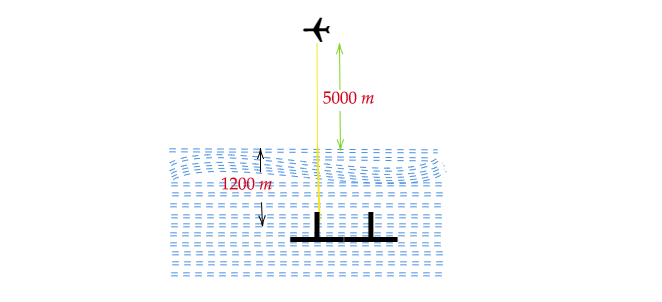# A plane is flying at a height of $5000\ m$ above sea level. At a particular point, it is exactly above a submarine floating $1200\ m$ below the sea level. What is the vertical distance between them?

Given:

A plane is flying at the height of $5000\ m$ above sea level.

At a particular point, it is exactly above a submarine floating $1200\ m$ below sea level.

To do:

We have to find the vertical distance between the plane and the submarine.

Solution:Height of the plane from the sea level $=5000\ m$

Depth of the submarine below the sea level $=1200\ m$

Therefore, the vertical distance between the plane and the submarine $=5000+1200$

$=6200\ m$

Hence, the vertical distance between the plane and the submarine is $6,200\ m$.